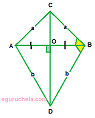# Kite Area and Perimeter Calculator

Calculator calculates the area of a kite with both methods (Trigonometry and Diagonal) and also calculate the perimeter of the kite.

Area of Kite [Diagonal Method] :

[ ½×d1d2 ]

 Enter Diagonal lengths ,

 Area of Kite =

Area of Kite Using Trigonometry:
[ ab Sin C ]

 Enter the lengths : ,
 Enter the angle :

 Area of Kite =

Perimeter of Kite:
[ 2(a+b) ]

 Enter the lengths : ,

 Perimeter of Kite =

## Formula

Perimeter of Kite:
[ 2(a+b) ]

Area of Kite Using Trigonometry:
[ ab Sin C ]

Area of Kite [Diagonal Method]:
[ ½×d1d2 ]The kite is a quadrilateral with two pairs of equal-length sides, adjacent to each other.

Kite is a symmetric shape and its diagonals are perpendicular.

There is two basic kite area formulas can be used depending on the information availble:

### Trigonometry method:

Area of the kite = product of both side length sin (angle between them) = ab sin C, Where a and b are the lengths of two unequal sides, C is the angle

### Diagonal method:

Area of the kite = The area is half the product of the diagonals = (d1d2)/2 where d1,d2 are the length of diagonal.

### Perimeter:

add the length of each side = 2a+2b Where a and b are the lengths each sides.

### Important properties of the kites

Two pairs of adjacent sides are equal (AD = BD and AC = BC).

One pair of opposite angles are equal (∠OBC = ∠OBD)

The sum of the interior angles of a kite is equal to 360°.

The diagonals of a kite are perpendicular to each other.

The longer diagonal of the kite bisects the shorter diagonal.

The area of a kite is equal to half of the product of the length of its diagonals.

The perimeter of a kite is equal to the sum of the length of all of its sides.